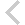FICO Xpress Optimization Examples Repository
 FICO Optimization Community FICO Xpress Optimization HomeFolio - Modelling examples from 'Getting started'

Description
• Chapter 3 Inputting and Solving a Linear Programming problem
• foliolp.mos: modeling and solving a small LP problem
• foliolperr.mos: LP model with syntax errors
• foliolps.mos: LP model using string indices
• Chapter 4 Working with data
• foliodata.mos (data file: folio.dat): data input from file, result output to a file, model parameters
• folioodbc.mos (data files: folio.xls, folio.mdb, folio.sqlite): data input from a spreadsheet or database, result output to a spreadsheet or database, model parameters
• folioexcel.mos (data file: folio.xls): same as folioodbc.mos but with Excel-specific data input and output (Windows only)
• foliosheet.mos (data file: folio.xls): same as folioodbc.mos but with data input and output through generic spreadsheet access
• foliocsv.mos (data file: folio.csv): same as folioodbc.mos but with data input and output through generic spreadsheet access in CSV format
• Chapter 5 Drawing user graphs
• folioloop.mos (data files: folio.dat, foliodev.dat): re-solving with varied parameter settings
• folioloop_graph.mos (data files: folio.dat, foliodev.dat): re-solving with varied parameter settings, graphical solution display
• foliolps_graph.mos: same as foliolps, adding graphical solution display
• Chapter 6 Mixed Integer Programming
• foliomip1.mos (data file: folio.dat): modeling and solving a small MIP problem (binary variables)
• foliomip2.mos (data file: folio.dat): modeling and solving a small MIP problem (semi-continuous variables)
• folioqp.mos (data file: folioqp.dat): modeling and solving a QP and a MIQP problem
• folioqp_graph.mos (data files: folioqp.dat, folioqpgraph.dat): re-solving a QP problem with varied parameter settings, graphical solution display
• folioqc.mos (data file: folioqp.dat): modeling and solving a QCQP and
• foliomiqc.mos (data file: folioqp.dat): modeling and solving a MIQCQP
• Chapter 8 Heuristics
• folioheur.mos (data file: folio.dat): heuristic solution of a MIP problem

Source Files

Data Files

folioqp.mos

```(!******************************************************
Mosel Example Problems
======================

file folioqp.mos
````````````````
Modeling a small QP problem
to perform portfolio optimization.
-- 1. QP: minimize variance
2. MIQP: limited number of assets ---

(c) 2008 Fair Isaac Corporation
author: S.Heipcke, Aug. 2003, rev. Sep. 2017
*******************************************************!)

model "Portfolio optimization with QP/MIQP"
uses "mmxprs", "mmnl"

parameters
MAXVAL = 0.3                      ! Max. investment per share
MINAM = 0.5                       ! Min. investment into N.-American values
MAXNUM = 4                        ! Max. number of different assets
TARGET = 9.0                      ! Minimum target yield
end-parameters

declarations
SHARES = 1..10                    ! Set of shares
RISK: set of integer              ! Set of high-risk values among shares
NA: set of integer                ! Set of shares issued in N.-America
RET: array(SHARES) of real        ! Estimated return in investment
VAR: array(SHARES,SHARES) of real ! Variance/covariance matrix of
! estimated returns
end-declarations

initializations from "folioqp.dat"
RISK RET NA VAR
end-initializations

declarations
frac: array(SHARES) of mpvar      ! Fraction of capital used per share
end-declarations

! **** First problem: unlimited number of assets ****

! Objective: mean variance
Variance:= sum(s,t in SHARES) VAR(s,t)*frac(s)*frac(t)

! Minimum amount of North-American values
sum(s in NA) frac(s) >= MINAM

! Spend all the capital
sum(s in SHARES) frac(s) = 1

! Target yield
sum(s in SHARES) RET(s)*frac(s) >=  TARGET

! Upper bounds on the investment per share
forall(s in SHARES) frac(s) <= MAXVAL

! Solve the problem
minimize(Variance)

! Solution printing
writeln("With a target of ", TARGET, " minimum variance is ", getobjval)
forall(s in SHARES) writeln(s, ": ", getsol(frac(s))*100, "%")

! **** Second problem: limit total number of assets ****

declarations
buy: array(SHARES) of mpvar       ! 1 if asset is in portfolio, 0 otherwise
end-declarations

! Limit the total number of assets
sum(s in SHARES) buy(s) <= MAXNUM

forall(s in SHARES) do